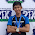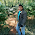## Electronic Devices Objective Questions

 For harmonic generation the amplifier used is
a)Audio Amplifier
b)Class-A Amplifier
c)RC Amplifier
d)class-C Turned Amplifier

 Zener diodes semiconductors are

a)Lightly Doped
b)Heavily Doped
c)Medium Doped
d)Not at all Doped

 In FET amplifiers, input is Incorrect!

a)A current signal
b)A voltage signal
c)Either a current or voltage signal
d)None of these

 H-parameters of a transistor

a)Are constant
b)Vary with temperature
c)Are dependent upon collector current
d)None of these

 In a voltage series feedback

a)Output resistance increases while input resistance decreases
b)Output and input resistances are reduced
c)Output and input resistances are increased
d)Output resistance decreases while input resistance increases

In a saturated transistor

a)B-E junction is forward biased with C-B junction is reverse biased
b)Both the junctions are forward biased
c)B-E junction is reverse biased with C-B junction is forward biased
d)Both the junctions are reverse biased

For an ideal noise free amplifier, the noise figure is

a)Zero
b)Zero dB
c)Infinity
d)1 dB

When a junction is formed between a metal and a semiconductor, the depletion layer is

a)More on the side of the metal
b)Equal on both sides
c)Less on the side of the metal
d)Less on semiconductor side

 The impurity commonly used for realizing the base region of a silicon NPN transistor is ______

A) Gallium
B) Indium
C) Boron
D) Phosphorus

 A MOS capacitor made using P-type substrate is in the accumulation mode. The dominant charge in the channel is due to the presence of

A) Holes
B) Electrons
C) Positively charged ions
D) Negatively charged ions

 A Zener diode works on the principle of ______

A) Tunneling of charge carriers across the junction
B) Thermionic emission
C) Diffusion of charge carriers across the junction
D) Hopping of charge carriers across the junction

 A BJT is said to be operating in the saturation region if______

A) Both the junctions are reverse biased
B) Base-emitter junction is reverse biased & base-collector junction is forward biased
C) Base-emitter junction is forward biased & base-collector junction is reverse biased
D) Both the junctions are forward biased

 To obtain very high input & output impedances in a feedback amplifier, the topology used is

A) Voltage-Series
B) Current-Series
C) Voltage-Shunt
D) Current-Shunt

 Cut off frequency of a bipolar transistor ____

A) Increase with the increase in base width
B) Increase with the increase in emitter width
C) Increase with the increase in the collector width
D) Increase with the decrease in the base width

 Negative feedback in amplifiers

A) Improves the signal to noise ratio at the input
B) Improves the signal to noise ratio at the output
C) Does not affect the signal to noise ratio at the output
D) Does not affect the signal to noise ratio at the input

9:35 AM

#### 6 comments:

1.Answer in question 5 must be (D). Series feedback increases input resistance and for voltage, as the sampled quantity from the output for feedback. Sampling connection is shunt so output resistance decreases.

2.On a similar note, answer in question 13 must be (B) current series.

3.4.Q NO 10 IS ANS IS (A) HOLES

5.question number 5 answer is D and question number 13 answer is B please update the answer

6.13 ans is current series feedback